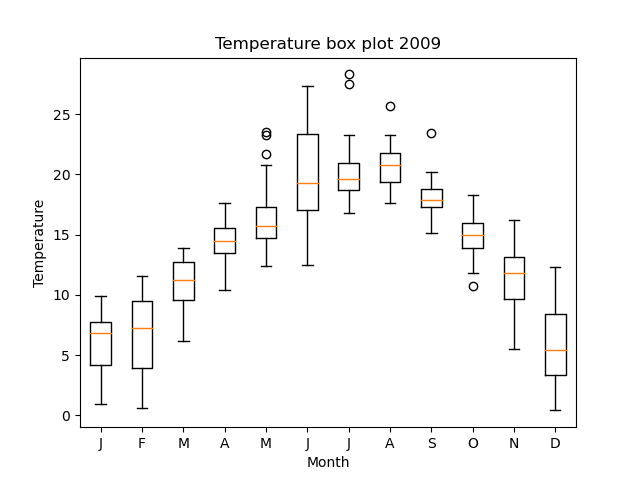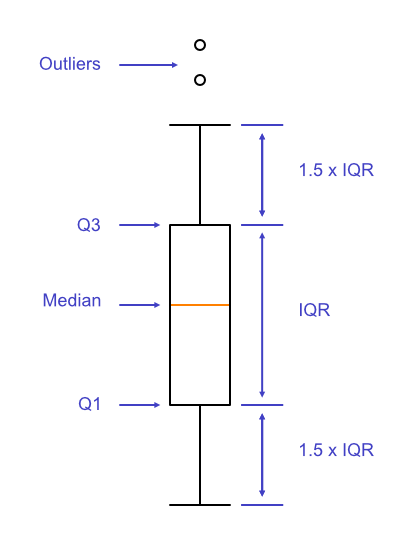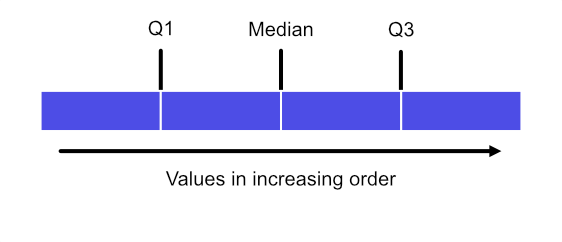# Box plots in Matplotlib

By Martin McBride, 2022-06-24
Tags: matplotlib box plot
Categories: matplotlibWe have previously used bar charts to show the average temperature of each month.

Box plots show the average temperature of each month, but they also indicate the spread of temperatures for each month.

## Example box plot

Here is a box plot of monthly temperatures for 2009:The bar and whisker figure for each month is based on the data for all the days in that month, so there are between 28 and 31 temperatures represented by each figure, depending on the month.

Here is one box in a bit more detail:The box is based on the quartiles of the data. To find the quartiles, we arrange the values of the data set in increasing order, then we divide the list into 4 equal parts:• The first quartile (Q1) is the upper boundary of the first group. A quarter of the values of the set are less than Q1.
• The median is the upper boundary of the second group. Half of the values of the set are less than the median. The median is also known as the second quartile (Q2).
• The third quartile (Q3) is the upper boundary of the third group. Three-quarters of the values of the set are less than Q3.

The box stretches from Q1 to Q3, with the median indicated by the orange line.

The interquartile range (IQR) is the distance between Q1 and Q3 (ie it is Q3 - Q1).

The lower whisker usually indicates the smallest data point. However, the length of the whisker is limited to 1.5 times the IQR. So it will indicate the smallest value that is not less than:

Q1 - 1.5 * IQR


Any points that are less than this value are called outliers and are indicated separately by small circles.

The upper whisker usually indicates the largest data point, and again the length of the whisker is limited to 1.5 times the IQR. So it will indicate the largest value that is not greater than:

Q3 + 1.5 * IQR


Any points that are greater than this value are also outliers, and again are indicated separately by small circles.

## Creating a simple box plot

Here is the code to create the plot above:

import matplotlib.pyplot as plt
import csv

with open("2009-temp-monthly-list.csv") as csv_file:

month_names = ["J", "F", "M", "A", "M", "J",
"J", "A", "S", "O", "N", "D"]

months = range(12)

plt.title("Temperature box plot 2009")
plt.xlabel("Month")
plt.ylabel("Temperature")
plt.boxplot(temperature, positions=months)
plt.xticks(months, month_names)
plt.show()


The code is here on github, in the file boxplot_temperatures.py.

This is quite similar to creating a bar chart.

The main difference is that we are reading in a list of lists, so we don't need to flatten the list. So we create the temperature list like this:

temperature = list(csv_reader)


This converts the csv_reader object (which is an iterator) into a list, without flattening. Here is the resulting list of lists:

[
[1.2, 3.8, 2.4, 1.7, ...] # 31 values for Jan
[2.7, 0.6, 2.6, ...]      # 28 values for Feb
[10.1, 10.3, 9.3, ... ]   # 31 values for Mar
...
]


The outer list contains 12 sublists, one for each month. Each sublist contains the temperatures for each day of that particular month, as described earlier.

We make the box plot using plt.boxplot. This accepts the list of lists, and also a positions parameter that we use to place the boxes at x positions 0, 1 ... 11.